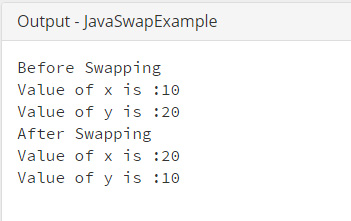## Java Programming Examples Tutorial Index

This Java program is used to swapping two numbers, without using a temporary variable.

Example:

``````public class JavaSwapExample {

public static void main(String[] args) {

int x = 10;
int y = 20;

System.out.println("Before Swapping");
System.out.println("Value of x is :" + x);
System.out.println("Value of y is :" + y);

//add both the numbers and assign it to first
x = x + y;
y = x - y;
x = x - y;

System.out.println("Before Swapping");
System.out.println("Value of x is :" + x);
System.out.println("Value of y is :" + y);
}
}
``````

Program Output:Explanation:

This program explains about how you can use the concept of swapping of values within a variable without using the third variable. Here third variable means, without using temporary variable. First of all you have to create a class having access specifier as 'public' and name 'JavaSwapExample'. Within this class, the main() method has been declared where two integer type variables 'x' and 'y' have been declared and initialized.

int x = 10;

int y = 20;

Now before swapping the values present in the variables are shown using the System.out.println(). Now, the trick for swapping two variable's values without using the temporary variable is that

x = x + y;

y = x - y;

x = x - y;

first variable is first added to the second variable and stored in first variable. Then the second variable is subtracted from first variable and stored in second variable. Lastly, the value of 2nd variable is subtracted from 1st and stored in first variable. This is how the values of one variable get swapped to another and vice versa, i.e. x becomes 20 and y becomes 10.

Finally, the swapped value gets printed using the System.out.println() method.